Mathematics Quiz

## Ted M. Montgomery, O.D.

After reading each question below, click on the ôpop-upö box after the question to activate it.  Then click on the answer you think is correct.  If you want to change an answer, click on that box again and select another choice.

If you are unsure of an answer, use a Search Engine to attempt to find the answer on the world wide web.  Then return back here and select your answer.

When you are done, click on the ôScore Quizö button at the bottom of the ôSummary of Answersö section.  This will show you how many you got correct!

 1.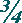Î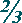=     ? 1/35/121/25/6 2.û=     ? 1/121/61/41/3 3.+=     ? 5/121/21 1/21 5/12 4.¸=     ? 5/121/21 1/121 1/8 5. What is the geometric term describing the relationship between triangles ABC and DEF?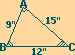analogouscongruentequivalentsimilar 6. Using the Pythagorean Theorem for right triangle ABC, if side a = 120 meters in length and side b = 119 meters in length, what is the length of side c?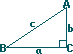124 meters169 meters194 meters239 meters 7. Consider this equation: ax▓ + bx + c = 0.  What is the name of the following formula, which is used to solve for x in that equation?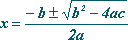coefficient formulaequilibrium formulaquadratic formulasquare root formula 8. How is this equation factored? x▓ + 4x û 12 = 0 (x - 2)(x + 6) = 0(x + 2)(x - 6) = 0(x - 3)(x + 4) = 0(x + 3)(x - 4) = 0 9.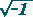or ôiö is not what? a complex numberan imaginary numbera rational number(0 , 1)  or  0 + 1i 10. For right triangle ABC, if a = 4 inches in length and b = 3 inches in length, what is the cosine of angle A?4/53/43/51 1/4

If there is any blank space under ôYour Answers,ö it means that you did not answer that question.  You can go back and make any changes in your answers before you click on the ôScore Quizö button, which will cause the right responses to appear under ôCorrect Answers.ö

1.
2.
3.
4.
5.
6.
7.
8.
9.
10.
1.
2.
3.
4.
5.
6.
7.
8.
9.
10.

You got

Return to TedÆs General Knowledge Quizzes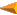Return to TedÆs HOMEPAGE